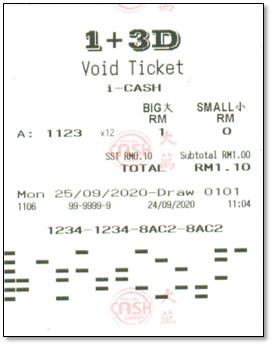Your lucky number for today 0000

# How To Play - 1+3D

For the Special Cash Sweep 1 + 3D Big or 1 + 3D Small, you can pick your choice of four 4-digit number from a range of 10,000 numbers between “0000” and “9999”. You will win a prize when your chosen number matches one of the 23 winning numbers drawn by us.

There are 8 ways to play:-
1.  1+3D Standard (Big & Small)

Select a 4-digit number within the range of “0000” and “9999”.2.  1+3D Lucky Pick

Allow the system to randomly generate a 4-digit number for you.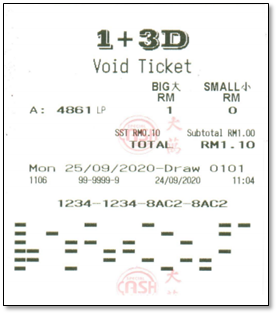3.  1+3D Permutation

Select a 4-digit number and let the system generates all possible number of permutations. The total possible number of digit permutations will be dependent on the selected 4-digit number. The possibilities are: -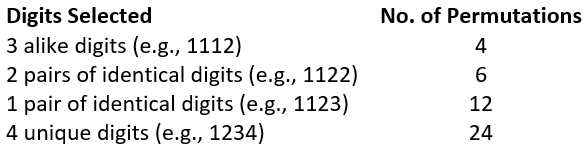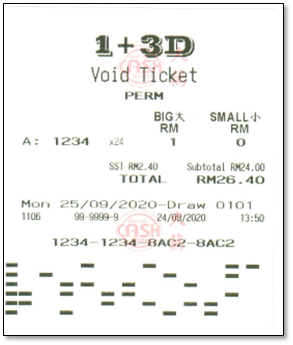4.  1+3D Roll First

Select your last 3 digits of a 4-digit number and let the system “roll” the first digit number from 0 to 9 (inclusive) for a total of ten 4-digit bets.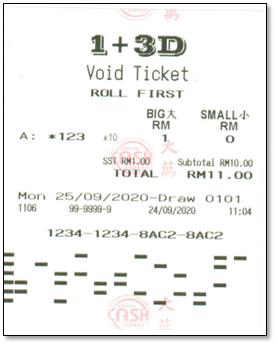5.  1+3D Roll Last

Select the first 3 digits of a 4-digit number and let the system “roll” the last digit number from 0 to 9 (inclusive) for a total of ten 4-digit bets.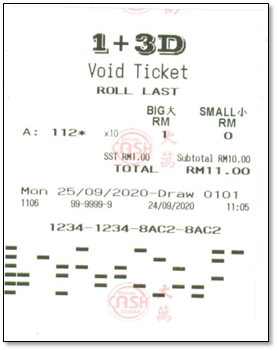6.  1+3D Perm Last 3

Select a 4-digit number and let the system generates a permutation of the last 3-digit number. The total possible number of digit permutations will be dependent on the last 3-digit number selected. The possibilities are: -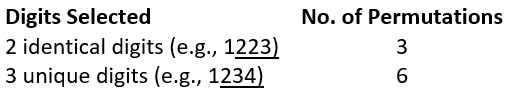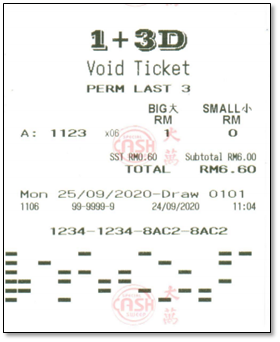7.  1+3D Perm Front 3

Select a 4-digit number and let the system generates a permutation of the first 3-digit number. The total possible number of digit permutations will be dependent on the first 3-digit number selected. The possibilities are: -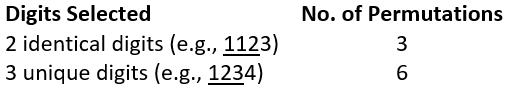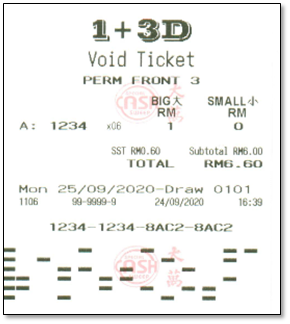8.  i-CASH

i-Cash allows the bet of all possible number of combinations based on a selected 4-digit number at RM1.00 per play.  The prize payout will be based on the calculation below: -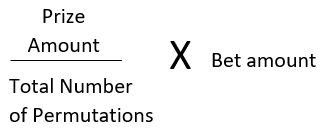Do take note that since the winning chances are greatly improved, the prize payout is also reduced accordingly.